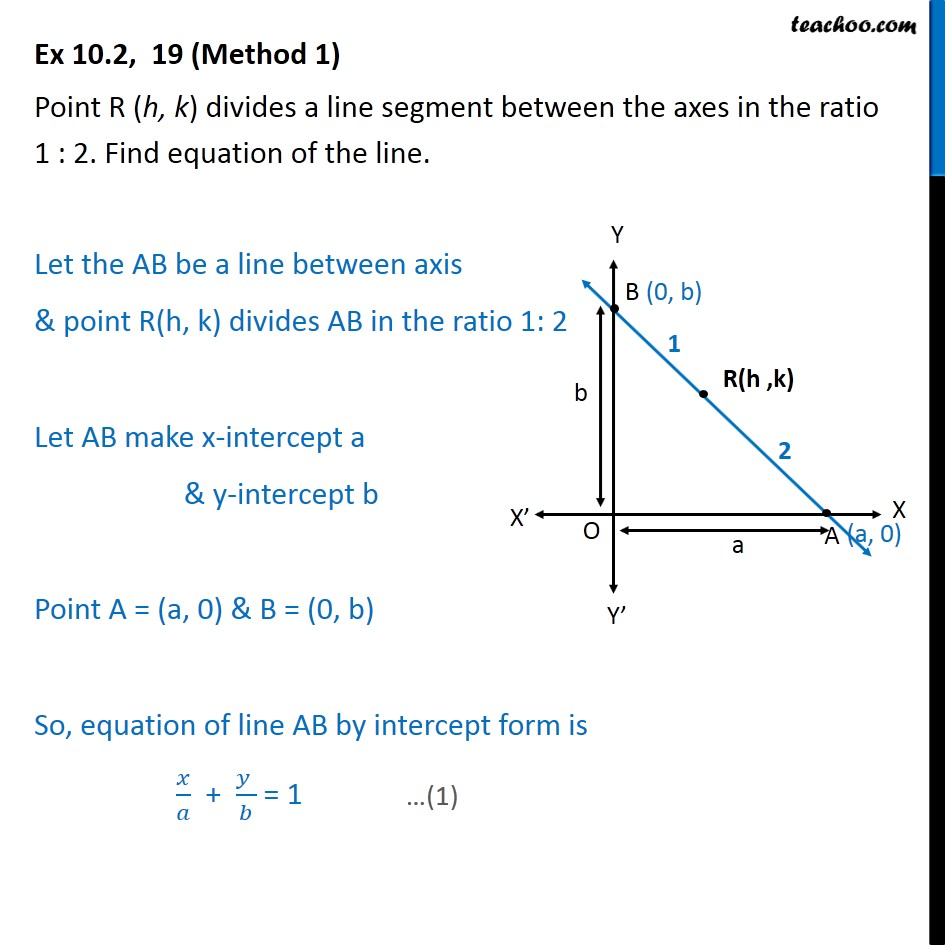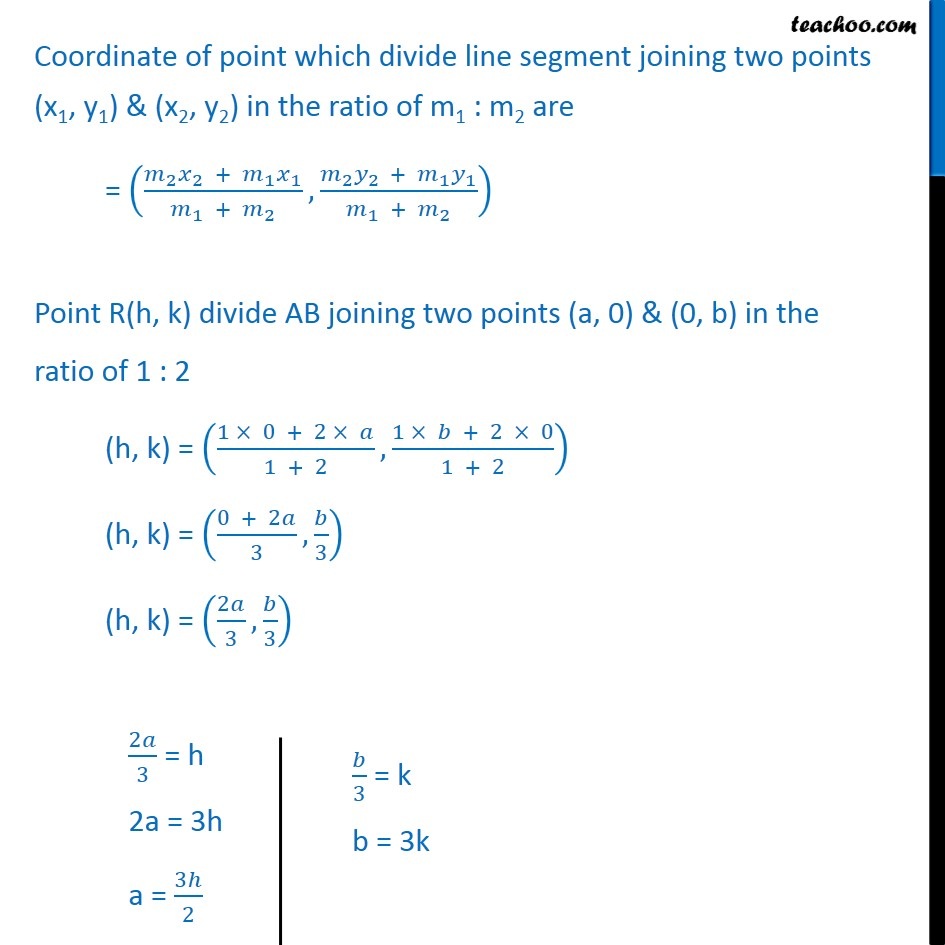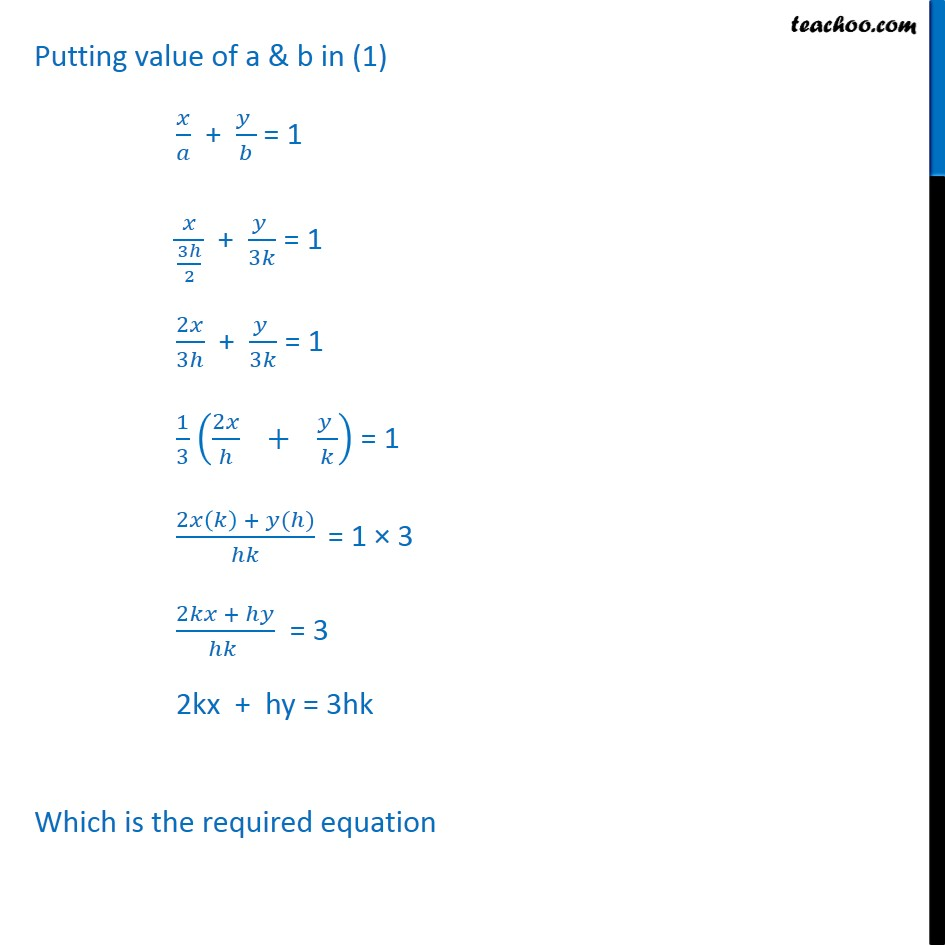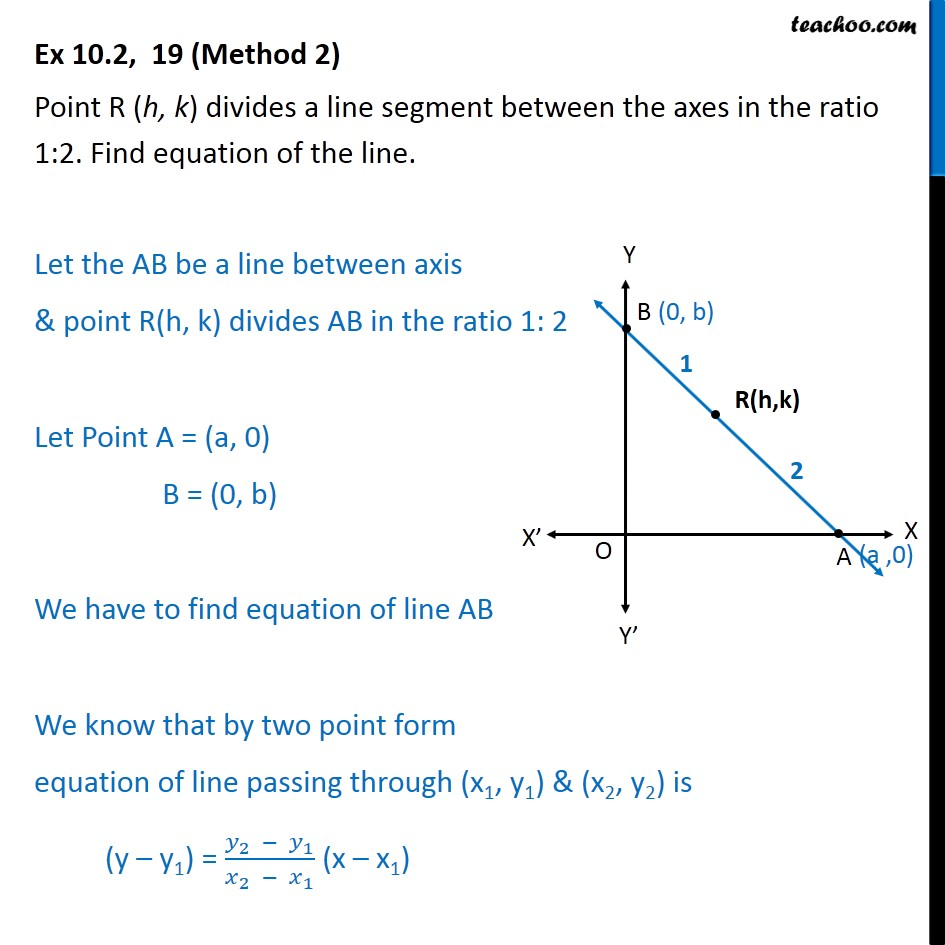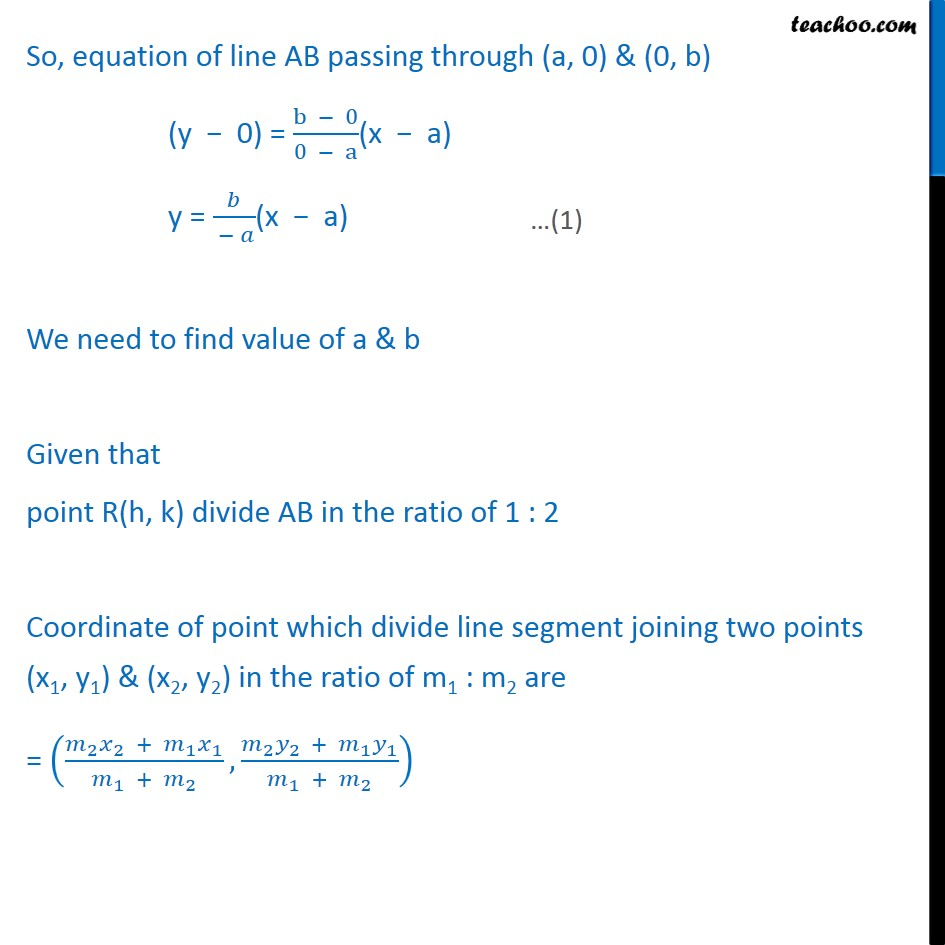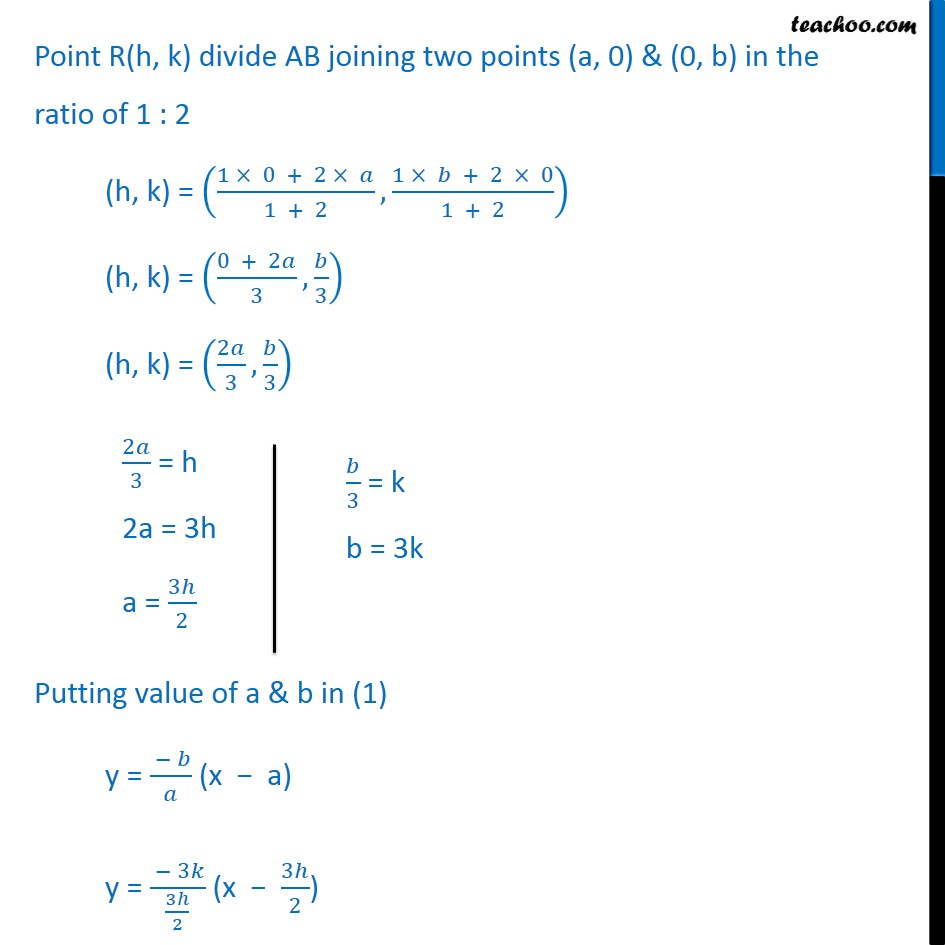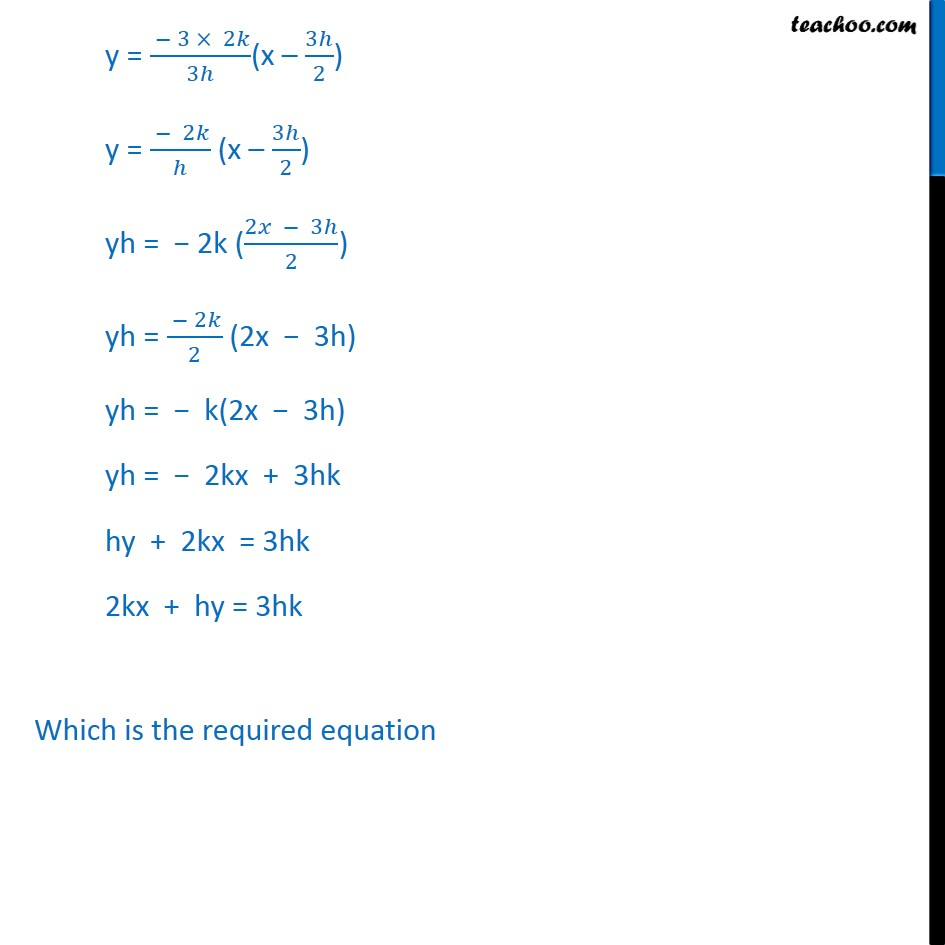1. Chapter 10 Class 11 Straight Lines
2. Serial order wise
3. Ex 10.2

Transcript

Ex 10.2, 19 (Method 1) Point R (h, k) divides a line segment between the axes in the ratio 1 : 2. Find equation of the line. Let the AB be a line between axis & point R(h, k) divides AB in the ratio 1: 2 Let AB make x-intercept a & y-intercept b Point A = (a, 0) & B = (0, b) So, equation of line AB by intercept form is 𝑥﷮𝑎﷯ + 𝑦 ﷮𝑏﷯ = 1 Coordinate of point which divide line segment joining two points (x1, y1) & (x2, y2) in the ratio of m1 : m2 are = 𝑚﷮2﷯ 𝑥﷮2﷯ + 𝑚﷮1﷯ 𝑥﷮1﷯﷮ 𝑚﷮1﷯ + 𝑚﷮2﷯﷯, 𝑚﷮2﷯ 𝑦﷮2 ﷯ + 𝑚﷮1﷯ 𝑦﷮1﷯﷮ 𝑚﷮1﷯ + 𝑚﷮2﷯﷯﷯ Point R(h, k) divide AB joining two points (a, 0) & (0, b) in the ratio of 1 : 2 (h, k) = 1 × 0 + 2 × 𝑎﷮1 + 2﷯, 1 × 𝑏 + 2 × 0﷮1 + 2﷯﷯ (h, k) = 0 + 2𝑎﷮3﷯, 𝑏﷮3﷯﷯ (h, k) = 2𝑎﷮3﷯, 𝑏﷮3﷯﷯ Putting value of a & b in (1) 𝑥﷮𝑎﷯ + 𝑦 ﷮𝑏﷯ = 1 𝑥﷮ 3ℎ﷮2﷯﷯ + 𝑦 ﷮3𝑘﷯ = 1 2𝑥﷮3ℎ﷯ + 𝑦 ﷮3𝑘﷯ = 1 1﷮3﷯ 2𝑥﷮ℎ﷯ + 𝑦 ﷮𝑘﷯﷯ = 1 2𝑥 𝑘﷯ + 𝑦(ℎ)﷮ℎ𝑘﷯ = 1 × 3 2𝑘𝑥 + ℎ𝑦﷮ℎ𝑘 ﷯ = 3 2kx + hy = 3hk Which is the required equation Ex 10.2, 19 (Method 2) Point R (h, k) divides a line segment between the axes in the ratio 1:2. Find equation of the line. Let the AB be a line between axis & point R(h, k) divides AB in the ratio 1: 2 Let Point A = (a, 0) B = (0, b) We have to find equation of line AB We know that by two point form equation of line passing through (x1, y1) & (x2, y2) is (y – y1) = 𝑦﷮2﷯ − 𝑦﷮1﷯﷮ 𝑥﷮2﷯ − 𝑥﷮1﷯﷯ (x – x1) So, equation of line AB passing through (a, 0) & (0, b) (y − 0) = b − 0﷮0 − a﷯(x − a) y = 𝑏﷮ − 𝑎﷯(x − a) We need to find value of a & b Given that point R(h, k) divide AB in the ratio of 1 : 2 Coordinate of point which divide line segment joining two points (x1, y1) & (x2, y2) in the ratio of m1 : m2 are = 𝑚﷮2﷯ 𝑥﷮2﷯ + 𝑚﷮1﷯ 𝑥﷮1﷯﷮ 𝑚﷮1﷯ + 𝑚﷮2﷯﷯, 𝑚﷮2﷯ 𝑦﷮2 ﷯ + 𝑚﷮1﷯ 𝑦﷮1﷯﷮ 𝑚﷮1﷯ + 𝑚﷮2﷯﷯﷯ Point R(h, k) divide AB joining two points (a, 0) & (0, b) in the ratio of 1 : 2 (h, k) = 1 × 0 + 2 × 𝑎﷮1 + 2﷯, 1 × 𝑏 + 2 × 0﷮1 + 2﷯﷯ (h, k) = 0 + 2𝑎﷮3﷯, 𝑏﷮3﷯﷯ (h, k) = 2𝑎﷮3﷯, 𝑏﷮3﷯﷯ Putting value of a & b in (1) y = − 𝑏﷮𝑎﷯ (x − a) y = − 3𝑘﷮ 3ℎ﷮2﷯﷯ (x − 3ℎ﷮2﷯) y = − 3 × 2𝑘﷮3ℎ﷯(x – 3ℎ﷮2﷯) y = − 2𝑘﷮ℎ﷯ (x – 3ℎ﷮2﷯) yh = − 2k ( 2𝑥 − 3ℎ﷮2﷯) yh = − 2𝑘﷮2﷯ (2x − 3h) yh = − k(2x − 3h) yh = − 2kx + 3hk hy + 2kx = 3hk 2kx + hy = 3hk Which is the required equation

Ex 10.2